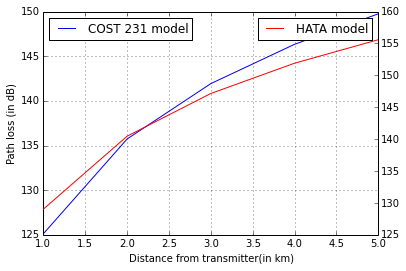# Chapter 3: Radio Propagation and Propagation Path-Loss Models¶

## Example 3.1, Page 51¶

In :
import math

#Variable declaration
hb=100; #in feets(height of BS antenna)
hm=5;  # in feets(height of mobile antenna)
f=881.52;#in MHz
lamda=1.116;  #in feet
d=5000;  #in feet
Gb=10**0.8;  #8dB(BS antenna gain)
Gm=10**0;   # 0dB (Mobile antenna gain)

#Calculations&Results
free_atten=(4*math.pi*d/lamda)**2*(Gb*Gm)**-1;
y=round(10*math.log10(free_atten));
print 'Free space attenuation is %d dB \n'%y
reflect_atten= (d**4/(hb*hm)**2)*(Gb*Gm)**-1;
x=round(10*math.log10(reflect_atten));
print 'Reflecting surface attenuation is %d dB \n '%x

Free space attenuation is 87 dB

Reflecting surface attenuation is 86 dB



## Example 3.2, Page 52¶

In :
import math

#Variable declaration
d=8000; #Distance between base station and mobile station
f=1.5*10**9;#in Hz
lamda=0.2;  #in metres
Pt=10; #BS transmitted power in watts
Lo=8;   #Total system losses in dB
Nf=5;    #Mobile receiver noise figure in dB
T=290;   #temperature in degree kelvin
BW=1.25*10**6; #in Hz
Gb=8;  #in dB
Gm=0;   #in dB
Hb=30;  #in metres
Hm=3.;   #in metres
B=1.38*10**-23; #Boltzmann's constant

#Calculations&Results
Free_Lp=20*math.log10(Hm*Hb/d**2);
Pr=Free_Lp-Lo+Gm+Gb+Pt;  #in dBW
Te=T*(3.162-1);
Pn=B*(Te+T)*BW;
print 'Received signal power is %d dBW \n'%(10*math.log10(Pn))
SNR=Pr-10*math.log10(Pn);
print 'SNR ratio is %d dB \n'%(round(SNR))

Received signal power is -138 dBW

SNR ratio is 31 dB



## Example 3.3, Page 58¶

In :
import math

#Variable declaration
d=3*1000;#in metres
Y=4;# path loss exponent
Pt=4;  #Transmitted power in watts
f=1800*10**6;#in Hz
d0=100.;#in metres
P0=-32;   #in dBm

#Calculations&Results
print "Using equation 3.11 and including shadow effect we get"
print 'Received power is %.1f dBm \n'%Pr
path_loss=10*math.log10(Pt*1000)-Pr;
print 'Allowable path loss is %.1f dB \n'%path_loss

Using equation 3.11 and including shadow effect we get

Allowable path loss is 116.6 dB



## Example 3.4, Page 58¶

In :
#Variable declaration
Lp=150;  #in dB

#Calculations&Results
print "Using equation given in Problem i.e Lp=133.2+40*math.log(d) we get,"
d=10**((Lp-10-133.2)/40);
print "Separation between transmitter and receiver as %.2f km"%d

Using equation given in Problem i.e Lp=133.2+40*math.log(d) we get,
Separation between transmitter and receiver as 1.48 km


## Example 3.5, Page 61¶

In :
import math

#Variable declaration
v=60*0.44704;  #.. mph to mps
fc=860*10**6;#in Hz
td=2*10**-6; #RMS delay spread in sec
c=3.*10**8;# speed of light in m/sec
Rs=19200.; #Coded symbol rate in bps

#Calculations&Results
lamda=c/fc;
fm=v/lamda; #Maximum doppler shift
tc=1/(2*math.pi*fm);#Channel coherence time
print 'Channel coherence time is %.4f sec \n'%tc
ts=1/Rs; #symbol interval
print 'Symbol interval is %d microsec \n'%(ts*10**6);
print "As the symbol interval is much smaller compared to the channel coherence time. So, Symbol distortion is minimal and fading is slow.";
print "";
Bc=1/(2*math.pi*td);
print 'Coherence Bandwidth is %.2f kHz \n'%(Bc/1000)

Channel coherence time is 0.0021 sec

Symbol interval is 52 microsec

As the symbol interval is much smaller compared to the channel coherence time. So, Symbol distortion is minimal and fading is slow.

Coherence Bandwidth is 79.58 kHz



## Example 3.6, Page 65¶

In :
import math

#Variable declaration
p=1;# reﬂection coefﬁcient of ground
c=3.*10**8;# velocity of light in free space(m/sec)
e=2.71828;#Euler's number
fm=20;  #in Hz
fc=900*10**6; #carrier frequency in Hz

#Calculations&Results
Nr=math.sqrt(2*math.pi)*fm*p*e**-(p**2);
print 'NO of fades per second are %.2f  \n'%Nr
Afd=e**-(p**2)/(p*fm*math.sqrt(2*math.pi));
print 'Average fade duration is %.4f sec \n '%Afd
v=fm*c/fc;
print 'Maximum velocity of mobile is %.2f m/sec = %d Km/hour \n'%(v,v*18/5);

NO of fades per second are 18.44

Average fade duration is 0.0073 sec

Maximum velocity of mobile is 6.67 m/sec = 24 Km/hour



## Example 3.7, Page 70¶

In :
import math
import matplotlib
import matplotlib.pyplot as plt
import numpy as np
%matplotlib inline

#Variable declaration
d=np.array([1, 2, 3, 4, 5]);  #in km
hb=30; #Height of BS antenna in metres
hm=2;# height of mobile antenna in matres
fc=900;#carrier frequency in MHz
W=15;  #street width(m)
b=30;  # distance between building along radio path (m)
phi=90; # incident angle relative to the street
hr=30; #in m

#Calculations
dellhm=hr-hm;
#L50=Lf+Lrts+Lms

# By COST 231 model
Lf=32.4+20*np.log10(d)+20*np.log10(fc);
L0=4-0.114*(phi-55);
Lrts=-16.9-10*math.log10(W)+10*math.log10(fc)+20*math.log10(dellhm)+L0;
Lbsh=-18*math.log10(11);
ka=54-0.8*hb;
dellhb=hb-hr;
kd=18-15*dellhb/dellhm;
kf=4+0.7*(fc/925-1);
Lms=Lbsh+ka+kd*np.log10(d)+kf*np.log10(fc)-9*np.log10(b);
L50=np.array([0, 0, 0, 0, 0])
L50=Lf+Lrts+Lms;

#Okumura/Hata model
ahm=(1.1*math.log10(fc)-0.7)*hm-(1.56*math.log10(fc)-0.8);
L_50=69.55+26.16*np.log10(fc)+(44.9-6.55*np.log10(hb))*np.log10(d)-13.82*np.log10(hb)-ahm;
L_50 = np.array(L_50)

#Results
fig,ax1 = plt.subplots()
ax1.plot(d,L_50,'b-')
ax1.set_xlabel('Distance from transmitter(in km)')
ax1.set_ylabel('Path loss (in dB)')
ax2 = ax1.twinx()
ax2.plot(d,L50,'r')
ax1.legend(['COST 231 model'],loc=0)
ax2.legend(['HATA model'],loc=0)
ax1.grid()
plt.show()
print "L50 values by Cost 231 model"
print '%.2f  %.2f  %.2f  %.2f  %.2f \n '%(L50,L50,L50,L50,L50);
print "L50 values bu Okumura/Hata model"
print '%.2f  %.2f  %.2f  %.2f  %.2f \n '%(L_50,L_50,L_50,L_50,L_50);
print "The results from the plot of two models shows that the calculated path loss with the COST 231 model is higher than the value obtained by the Okumura/Hata model."

#Answers vary due to built-in functions of Python usedL50 values by Cost 231 model
129.03  140.47  147.16  151.91  155.59

L50 values bu Okumura/Hata model
125.13  135.73  141.93  146.34  149.75

The results from the plot of two models shows that the calculated path loss with the COST 231 model is higher than the value obtained by the Okumura/Hata model.


## Example 3.8, Page 76¶

In :
import math

#Variable declaration
SNRmin=12;#in dB
n=3; #No of floors
Backgroundnoise=-115; #dBm
pt=100  #in dBm

#Calculations
pt_db=10*math.log10(pt);
Lpmax=pt_db-Sr;
#Refering table 3.4
Lp_d0=38;  #ref path loss at the first meter(dB)
Lf=15+4*(n-1); #signal attenuation through n floors
y=3; #path loss exponent
d=10**((Lpmax-Lp_d0-Lf-X)/30); #max allowable path loss

#Result
print 'Coverage radius of an access point = %d m \n'%(round(d))

Coverage radius of an access point = 54 m



## Example 3.9, Page 77¶

In :
import math

#Variable declaration
SSmean=-100; #signal strength(dBm)
sd=10;  #standard deviation(dB)

#Calculations
P_Smin=(0.5-0.5*math.erf((Sr-SSmean)/(math.sqrt(2)*sd)));

#Result
print 'probability of exceeding signal beyond the receiver sensitivity is %.2f \n'%(P_Smin)

probability of exceeding signal beyond the receiver sensitivity is 0.84



## Example 3.10, Page 81¶

In :
import math

#Variable declaration
Lp=140; # path losses in dB
k=1.38*10**-23; # Boltzmann’s constant (W/Kelvin-Hz)
k_db=10*math.log10(k);
f=900;#in MHz
Gt=8; #transmitting antenna gain(dB)
Ag=24;#gain of receiver ampliﬁer in dB
Nf=6;#Noise figure(dB)
L0=20; # other losses in dB
Lf=12; # antenna feed line loss in dB
T=24.6;#Temperature expressed in dB
R=39.8; # data rate in dB
Eb_No=10;#dB

#Calculations
#From equation  (3.54)
pt_db=M-Gt-Gr-Ag+ Nf + T+ k_db+ Lp+ Lf+ L0 + Fmargin+ R+ Eb_No;

Pt=10**(pt_db/10);  #dB into normal number

#Result
print 'Total transmitted power is %d Watts \n'%Pt

Total transmitted power is 6 Watts